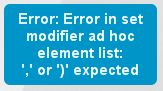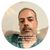# QlikView App Dev

Discussion Board for collaboration related to QlikView App Development.

Announcements
Support Cases coming to Qlik Community Oct. 4! Start chats, open cases, explore resources: READ DETAILS
cancel
Showing results for
Did you mean:Contributor III

## Max Year/Month available lower than selected filter period

Hi Masters

My date filter is:Variables:

vMaxDate = 01-Feb-2018    <==Max available date

vMaxYear = 2018                 <==Max available Year

vMaxMonth = Feb                <==Max available date

vFieldYear = 2018                <==Max selected year (filter)

vFieldMonthName = Dec     <==Max selected Month (filter)

Since we are now in February 2018, the maximum year and month available in my database is "2018" and "February".

I would like to write a script in a text box that would work like the following:

=if(Year>=\$(vMaxYear) and Month>\$(vMaxMonth),sum({1}{<Year={\$(=(\$(vMaxYear)))}, Month={\$(=(\$(vMaxMonth)))}>} NET_BAL),sum({1}{<Year={\$(=(\$(vFieldYear)))}, Month={\$(=(\$(vFieldMonthName)))}>} NET_BAL))

Basically, if the selected year and month (from the filter - 2018/Dec) are higher then the maximum year and month available (which is 2018 and Feb) than the disclosed result should be the max year/month available otherwise it should disclose the results for the selected period (filter).

Thanks

Felipe

1 Solution

Accepted SolutionsContributor III
Author

Thanks Digvijay!

At the end I made some changes, for example, the months variables are now showing the month number instead of month name.

vMaxMonth = 2

vFieldMonth = 2

After making those changes the query below worked as expected:

if(\$(vFieldYear)>=\$(vMaxYearDWH) and \$(=(\$(vFieldMonth#)))>\$(vMaxMonth#DWH),

num(sum({1}{<Year={\$(vMaxYearDWH)}, Month={\$(vMaxMonthDWH)}>} NET_BAL),'\$#,##0;(\$#,##0)'),

num(sum({1}{<Year={\$(=(\$(vFieldYear)))}, Month={\$(=(\$(vFieldMonthName)))}>} NET_BAL),'\$#,##0;(\$#,##0)')

13 RepliesSpecialist

I did not get well what you are looking for,

Let's try by fixing some syntax are you using {1} veriable? if not we can continue..

=if(Year>=\$(vMaxYear) and Month>\$(vMaxMonth),sum({<Year={\$(vMaxYear)},Month={\$(vMaxMonth)}>} NET_BAL),

,sum({<Year={\$(vFieldYear)}, Month={\$(vFieldMonthName)}>} NET_BAL))Contributor III
Author

Thanks Jahanzeb

I used this script:

=if(Year>=\$(vMaxYear) and Month>\$(vMaxMonth),sum({<Year={\$(vMaxYear)},Month={\$(vMaxMonth)}>} NET_BAL),sum({<Year={\$(vFieldYear)}, Month={\$(vFieldMonthName)}>} NET_BAL))

but I am getting the error below:MVP

May be this -

=if(Year>=\$(vMaxYear) and Month>\$(vMaxMonth),sum({1<Year={\$(vMaxYear)}, Month={\$(vMaxMonth)}>} NET_BAL),sum({<Year={\$(vFieldYear)}, Month={\$(vFieldMonthName)}>} NET_BAL)Contributor III
Author

it is missing a ")" at the end. You need to close the "if" and "sum" statementsSpecialist

yap you are right!MVP

My bad, after ')' is it giving the required output?Contributor III
Author

I get the same error aboveSpecialist

try this one , was extra

=if(Year>=\$(vMaxYear) and Month>\$(vMaxMonth),sum({<Year={\$(vMaxYear)},Month={\$(vMaxMonth)}>} NET_BAL)

,sum({<Year={\$(vFieldYear)}, Month={\$(vFieldMonthName)}>} NET_BAL))Contributor III
Author

This is the script I posted on my first reply to you. It didn't work.Community Browser